# What is multiplication? Multiplication as repeated addition

How much is 4 times 4?

Let’s take 4 multiplied by 4. The first number is the number of donuts in each group. We have four donuts in each group, and the second number is the number of groups. We have four groups of four donuts. that’s four plus four plus four plus four which makes sixteen donuts. So four times four is 16.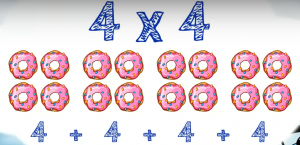## What is multiplying?

Multiplication is something you do with numbers like addition or subtraction. Multiplication is just repeating addition and nothing more. It’s represented by the × simple. Let’s say we want to multiply 3 by 4. The first number is the number we should add and the second number is the number of times we should add the first number.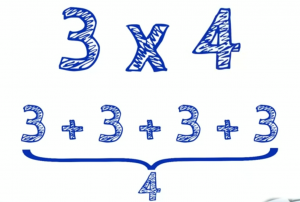In this example the first number is three. So we’ll add three and the second number is four. That means we’ll add three four times. So 3 plus 3 plus 3 plus 3 makes 12. That means 3 x 4 is 12.

Let’s take one more example. 5 x 3. The first number is 5. So we’ll add 5. The second number is 3. So we’ll need to add 5, 3 times. Then that is 5 plus 5 plus 5 which makes 15. The answer is 15.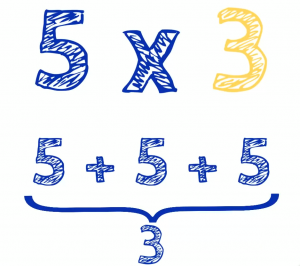You can prefer the first way with imagining sweets. Using just numbers looks a little bit boring, and you can prefer second way because it doesn’t make think about candy. You can use any approach you like. It doesn’t matter. The answer is the same.

Let’s try to solve another problem to make sure. How about 2 times 4. We solve choosing cake the first number is the number of pieces of cake in each group. There are two pieces of cake in each group. The second number is the number of groups. There are 4 groups of 2 pieces of cake and that’s 2 plus 2 plus 2 plus 2 pieces of cake in all. So 2 times 4 is 8.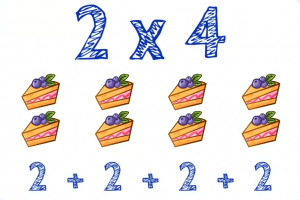Now it’s your turn. Multiply 2 by 4. The first number is the number we will be adding. The second number is the number of times we will addit. So we need to add 2 four times. That’s 2 plus 2 plus 2 plus 2. So 2 times 4 is 8.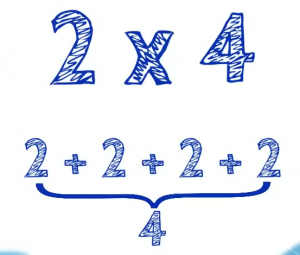See, we got the same answer. You can think of multiplication as groups of objects or just extended addition. It doesn’t matter which approach you use.